﻿ Using the Matrix Summability Method to Approximate the Lip (ξ(t), p) Class Functions

Using the Matrix Summability Method to Approximate the Lip (ξ(t), p) Class FunctionsOPEN ACCESSPEER-REVIEWED

Using the Matrix Summability Method to Approximate the Lip (ξ(t), p) Class Functions

Binod Prasad DhakalCentral Department of Education (Mathematics), Tribhuvan University, Nepal

Abstract

Most of the summability methods are derived from the matrix means. In this paper, author has been determined the degree of approximation of certain trigonometric functions belonging to the Lip (ξ(t), p) class by matrix method.

• Dhakal, Binod Prasad. "Using the Matrix Summability Method to Approximate the Lip (ξ(t), p) Class Functions." Turkish Journal of Analysis and Number Theory 2.6 (2014): 198-201.
• Dhakal, B. P. (2014). Using the Matrix Summability Method to Approximate the Lip (ξ(t), p) Class Functions. Turkish Journal of Analysis and Number Theory, 2(6), 198-201.
• Dhakal, Binod Prasad. "Using the Matrix Summability Method to Approximate the Lip (ξ(t), p) Class Functions." Turkish Journal of Analysis and Number Theory 2, no. 6 (2014): 198-201.

 Import into BibTeX Import into EndNote Import into RefMan Import into RefWorks

1. Introduction

The Fourier series associate with f at point x of 2π periodic function inis given by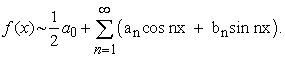(1.1)

A function f ∈Lip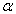if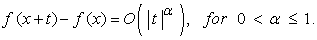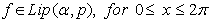, iffor 0 < α ≤ 1 and an integer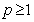.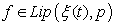if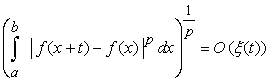provided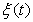is positive increasing function.

If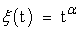then Lipcoincide with Lip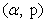and if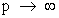in Lipthan Lipreduce to Lip α.

We observed that Lip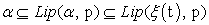for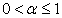.

We define norm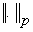by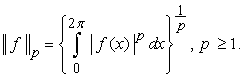(1.2)

The degree of approximation En (f) is given by(1.3)

where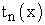is a trigonometric polynomial of degree n.

Let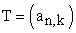be an infinite lower triangular matrix satisfying the condition(see, ) of regularity. Let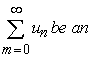infinite series such that whose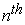partial sum s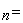.

The sequence-to-sequence transformationdefines the sequenceof lower triangular matrix means of the sequence {sn} generated by the sequence of coefficients (an,k).

The series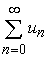is said to be summable to the sum s by lower triangular matrix method (see, ) if.

In this paper, we use following notations.(1.4)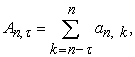(1.5)

where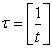is the greatest integer not greater than (1/t) and(1.6)

2. Main Theorem

Chandra proved a theorem on the approximation of function belonging to Lipclass by Nörlund and Riesz means. Mittal et. al.  extended the result of Chandra  by using the matrix means on same Lipclass function. In , Lal & Dhakal proved a theorem on approximation of a Lipclass function by matrix means.

Aim of this paper is to extend the theorems of Chandra , Mittal et.al.  and Lal & Dhakal  by using matrix means on Lipclass functions as following way:

Theorem. Let T=(an.k) be an infinite regular lower triangular matrix such that the element (an.k) be non-negative, non-decreasing with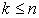. If a function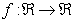is 2π-periodic, Lebesgue integrable on [-π, π] and belonging to Lip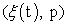class then the degree of approximation of f by lower triangular matrix means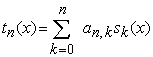of its Fourier series (1.1) satisfies, for n= 0,1,2,3…,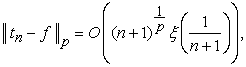(2.1)

provided ξ (t) satisfies the following conditions: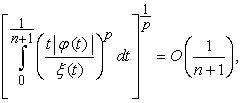(2.2)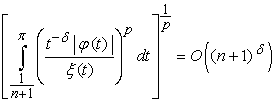(2.3)

where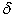is an arbitrary number such that, q the conjugate index of p and conditions (2.2) & (2.3) hold uniformly in x.

3. Lemmas

For the proof of the theorem following lemmas are required.

Lemma 1. Mn (t) = O (n +1), if.

Proof. For,,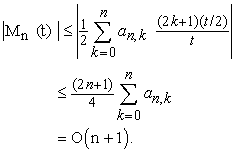Lemma 2. If (an,k) is non- negative and non- decreasing with, then,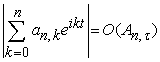, uniformly in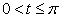.

Proof: Let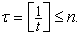Then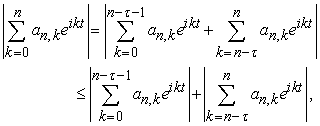by Abel’s lemma,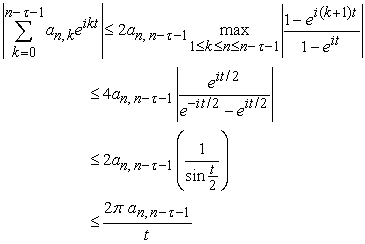andthereforeAlso,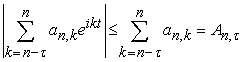.

ThusLemma 3., ifProof: For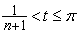, sin(t/2),we have4. Proof of the Theorem

Following , we havethen,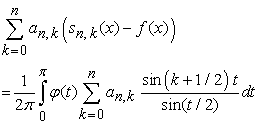or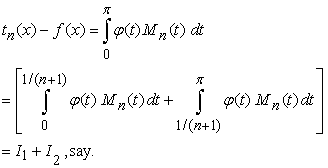(4.1)

For, I1,

Applying Holder’s inequality and fact that, we have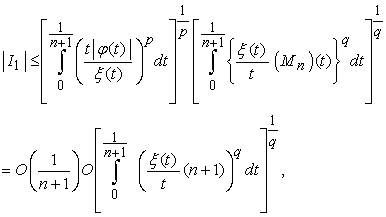by condition (2.2) & Lemma 1.

Applying Second Mean Value Theorem for Integrals, we have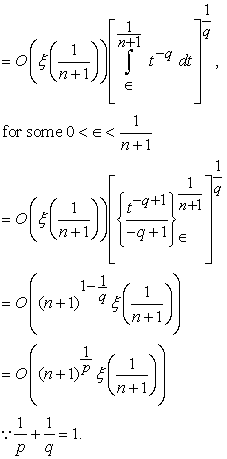(4.2)

Applying Holder’s inequality, condition (2.3) and Lemma 2.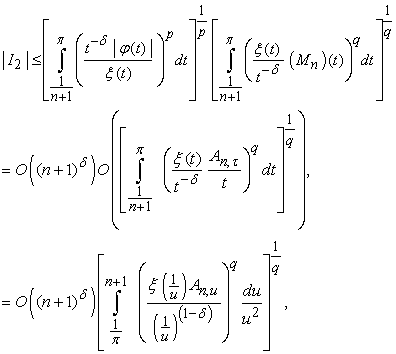taking(4.3)

Combining the conditions (4.1) – (4.3), we haveNow,(4.4)

This completes the proof of the theorem.

5. Corollaries

Following corollaries can be derived from the theorem.

Corollary 1. Ifthen degree of approximation of a function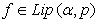class is given by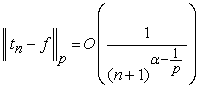forCorollary 2. Ifwith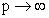, then degree of approximation of a function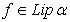class is given by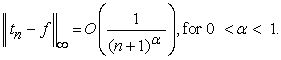References

  A. Zygmund, Trigonometric series, Cambridge University Press, 1959.In article  E. C. Titchmarsh, Theory of functions, Oxford University Press, 1939.In article  M. L Mittal, B. E. Rhoades, V. N. Mishra and U. Shing, Using infinite matrices to functions of class Lip (α,p) using trigonometric polynomials, J. Math. Anal. Appl, 326(2007), 667-676.In article CrossRef  O.Töeplitz, Über allgemeine lineare Mittelbildungen, Prace mat. - fiz., 22(1913), 113-119.In article  P. Chanrda, Trigonometric approximation of function in Lp-norm, J. Math. Anal. Appl, 275(2002), 13-676.In article CrossRef  S. Lal and B. P. Dhakal, On Approximation of functions belonging to Lipschitz class by triangular matrix method of Fourier series, Int. Journal of Math. Analysis, 4(21), 2010, 1041-1047.In article